﻿ Java exercises: Display the current date and time - w3resource# Java Method Exercises: Display the current date and time

## Java Method: Exercise-15 with Solution

Write a Java method to display the current date and time.

Pictorial Presentation: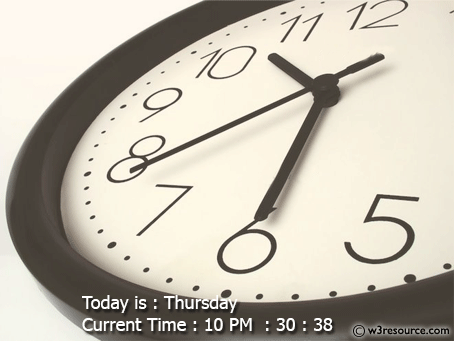Sample Solution:

Java Code:

``````import java.util.Scanner;
public class Exercise15 {

public static void main(String[] args) {

// Obtain the total milliseconds since midnight, Jan 1, 1970
long totalMilliseconds = System.currentTimeMillis();

// Obtain the total seconds since midnight, Jan 1, 1970
long totalSeconds = totalMilliseconds / 1000;

// Compute the current second in the minute in the hour
long currentSecond = (int)(totalSeconds % 60);

// Obtain the total minutes
long totalMinutes = totalSeconds / 60;

// Compute the current minute in the hour
long currentMinute = (int)(totalMinutes % 60);

// Obtain the total hours
long totalHours = totalMinutes / 60;

// Compute the current hour
long currentHour = (int)(totalHours % 24);

long totalDays = totalHours / 24;

// current year
int currentYear = (int)(totalDays / 365) + 1970;

long daysInCurrentYear = (totalDays - numberOfLeapYearsSince1970(currentYear)) % 365;
if (currentHour > 0) daysInCurrentYear++; // add today

// get current month number
int currentMonthNum = getMonthFromDays(currentYear, (int) daysInCurrentYear);

// getting current month name
String month = getMonthName(currentMonthNum);

// getting day of current month
int daysTillCurrentMonth = getNumOfDaysToReachThatMonth(currentYear, currentMonthNum);

int startDay = getStartDay(currentYear, currentMonthNum);
int today = (int) daysInCurrentYear - daysTillCurrentMonth;
String dayOfWeek = dayNameOfWeek( ((startDay + today) % 7));

// Display results
System.out.println("Current date and time: " + dayOfWeek + " " + month + " " + today +", " + currentYear
+" " + currentHour + ":"
+ currentMinute + ":" + currentSecond );

}

public static String dayNameOfWeek(int dayOfWeek) {

switch (dayOfWeek) {
case 2: return "Monday";
case 3: return "Tuesday";
case 4: return "Wednesday";
case 5: return "Thursday";
case 6: return "Friday";
case 7: return "Saturday";
case 1: return "Sunday";
default: return null;
}
}

public static int numberOfLeapYearsSince1970(long year) {

int count = 0;
for (int i = 1970; i <= year; i++) {
if (isLeapYear(i))count++;
}
return count;
}

public static int getMonthFromDays(int year, int days) {
int dayTracker = 0;
for (int i = 1; i <= 12; i++) {

dayTracker += getNumberOfDaysInMonth(year, i);

if (dayTracker > days) return i;
}
return 12;
}

public static int getNumOfDaysToReachThatMonth(int year, int month) {
int dayTracker = 0;
for (int i = 1; i < month; i++) {

dayTracker += getNumberOfDaysInMonth(year, i);

}
return dayTracker;
}

/** Get the start day of month/1/year */
public static int getStartDay(int year, int month) {
final int START_DAY_FOR_JAN_1_1800 = 3;
// Get total number of days from 1/1/1800 to month/1/year
int totalNumberOfDays = getTotalNumberOfDays(year, month);

// Return the start day for month/1/year
return (totalNumberOfDays + START_DAY_FOR_JAN_1_1800) % 7;
}

/** Get the total number of days since January 1, 1800 */
public static int getTotalNumberOfDays(int year, int month) {
int total = 0;

// Get the total days from 1800 to 1/1/year
for (int i = 1800; i < year; i++)
if (isLeapYear(i))
total = total + 366;
else
total = total + 365;

// Add days from Jan to the month prior to the calendar month
for (int i = 1; i < month; i++)
total = total + getNumberOfDaysInMonth(year, i);

}

/** Get the number of days in a month */
public static int getNumberOfDaysInMonth(int year, int month) {

if (month == 1 || month == 3 || month == 5 || month == 7 ||
month == 8 || month == 10 || month == 12)
return 31;

if (month == 4 || month == 6 || month == 9 || month == 11)
return 30;

if (month == 2) return isLeapYear(year) ? 29 : 28;

return 0; // If month is incorrect
}

/** Determine if it is a leap year */
public static boolean isLeapYear(int year) {
return year % 400 == 0 || (year % 4 == 0 && year % 100 != 0);
}

/** Get the English name for the month 1-12 */
public static String getMonthName(int month) {
String monthName = "";
switch (month) {
case 1: monthName = "January"; break;
case 2: monthName = "February"; break;
case 3: monthName = "March"; break;
case 4: monthName = "April"; break;
case 5: monthName = "May"; break;
case 6: monthName = "June"; break;
case 7: monthName = "July"; break;
case 8: monthName = "August"; break;
case 9: monthName = "September"; break;
case 10: monthName = "October"; break;
case 11: monthName = "November"; break;
case 12: monthName = "December";
}

return monthName;
}

}
```
```

Sample Output:

```Current date and time: Thursday June 22, 2017 7:5:36
```

N.B.: The result may varry for your system date and time.

Flowchart: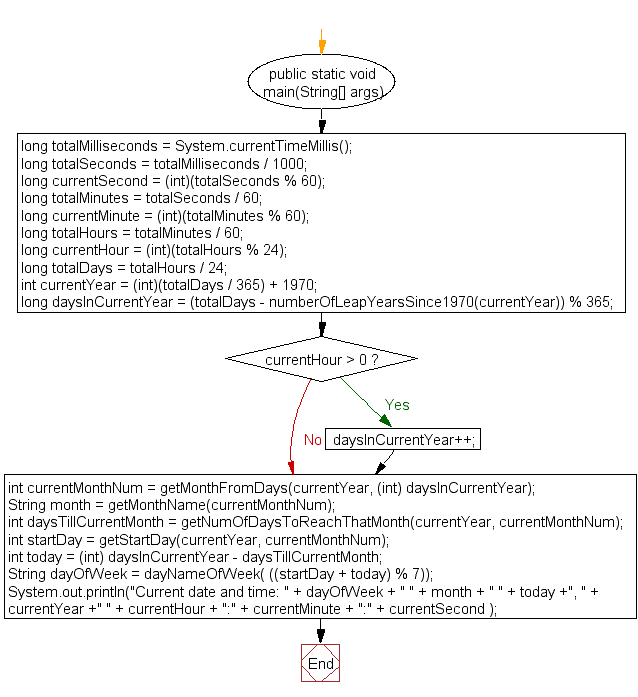Flowchart: dayNameOfWeek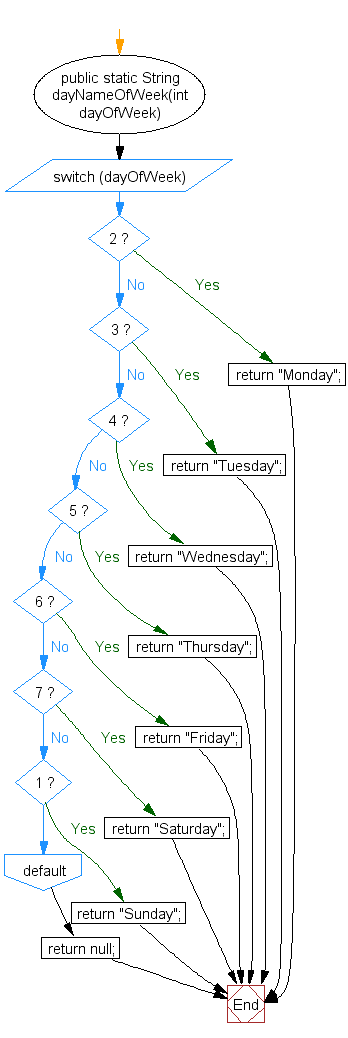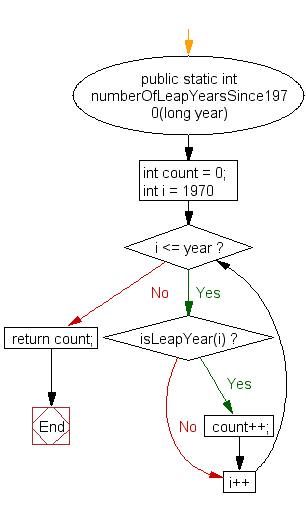Flowchart: getMonthFromDays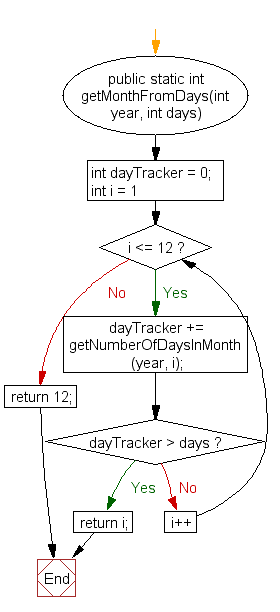Flowchart: getNumOfDaysToReachThatMonth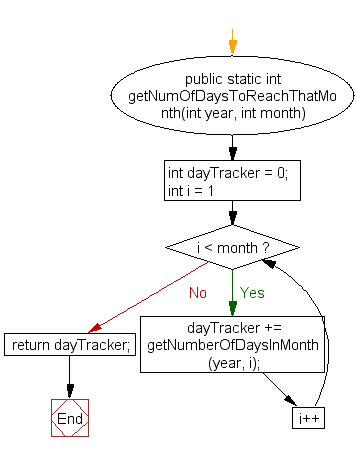Flowchart: getStartDay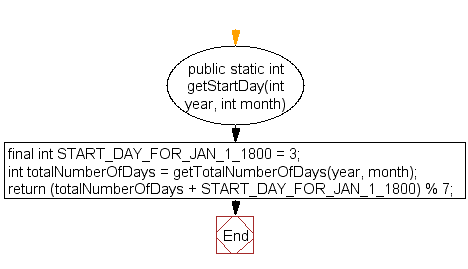Flowchart: getTotalNumberOfDays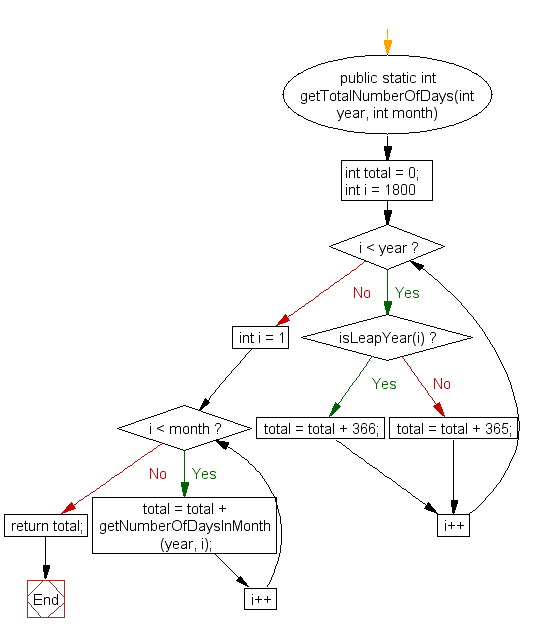Flowchart: getNumberOfDaysInMonth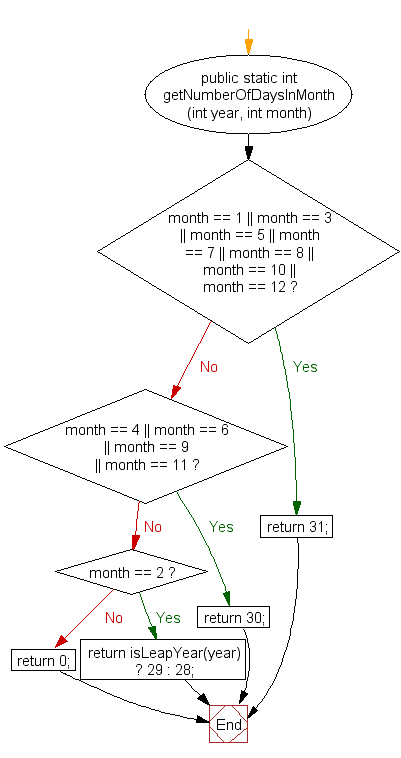Flowchart: isLeapYear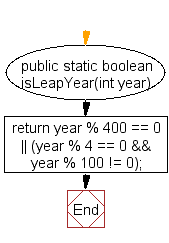Flowchart: getMonthName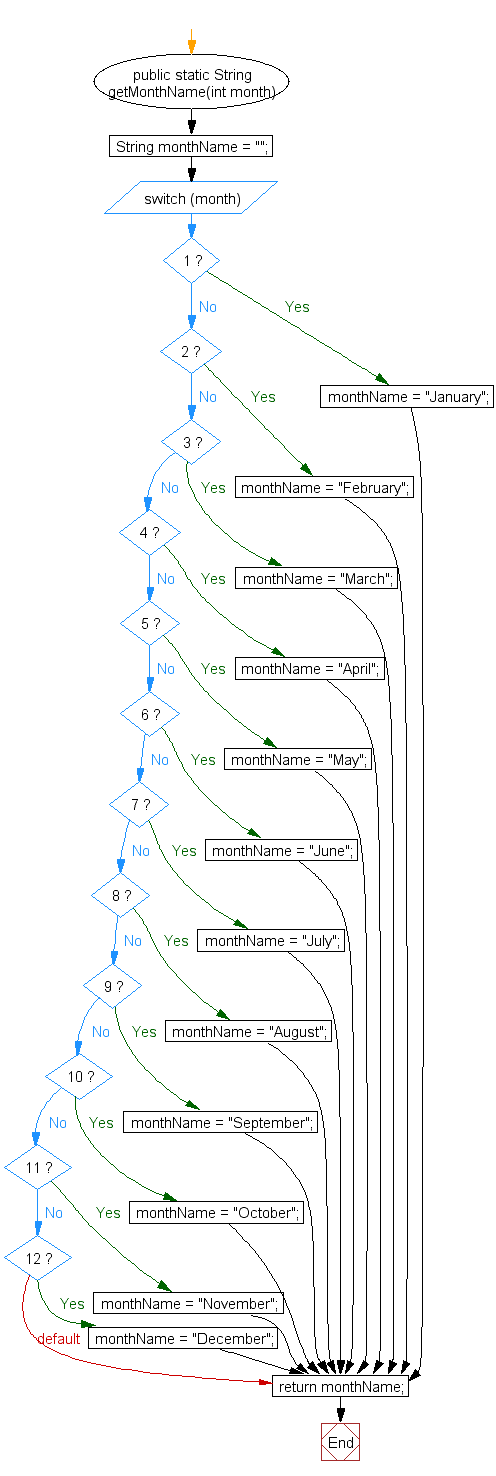Java Code Editor:

What is the difficulty level of this exercise?

﻿

## Java: Tips of the Day

Array vs ArrayLists:

The main difference between these two is that an Array is of fixed size so once you have created an Array you cannot change it but the ArrayList is not of fixed size. You can create instances of ArrayLists without specifying its size. So if you create such instances of an ArrayList without specifying its size Java will create an instance of an ArrayList of default size.

Once an ArrayList is full it re-sizes itself. In fact, an ArrayList is internally supported by an array. So when an ArrayList is resized it will slow down its performance a bit as the contents of the old Array must be copied to a new Array.

At the same time, it's compulsory to specify the size of an Array directly or indirectly while creating it. And also Arrays can store both primitives and objects while ArrayLists only can store objects.

Ref: https://bit.ly/3o8L2KH